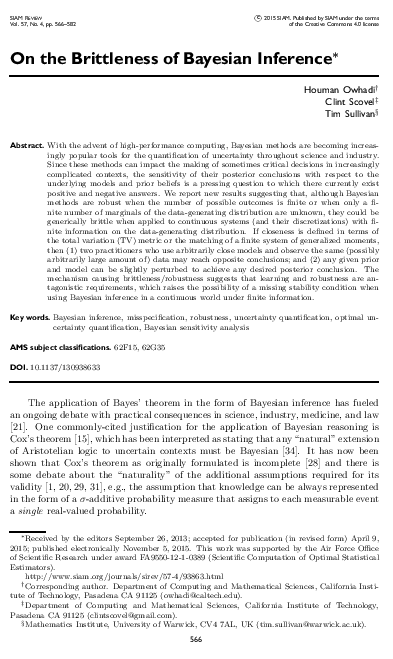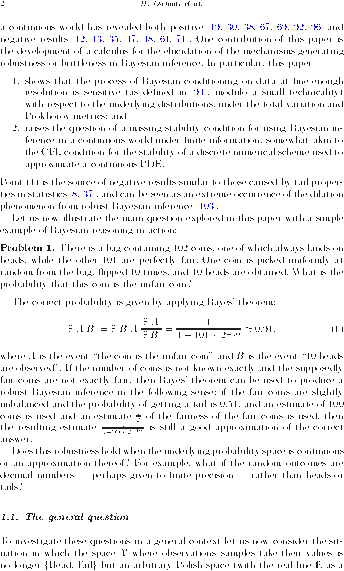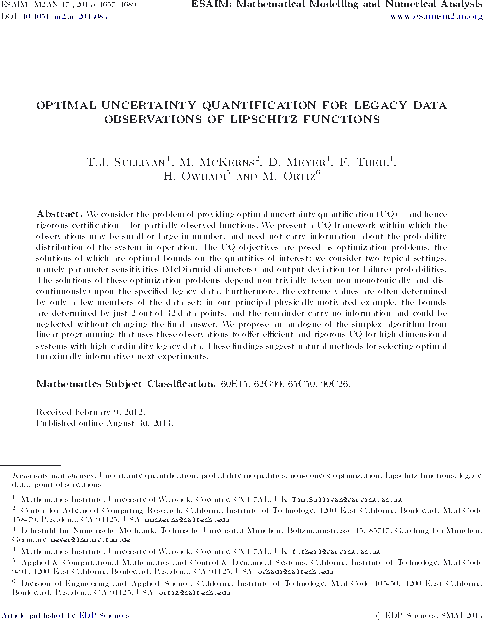# Tim Sullivan

Clear Search### Computing with dense kernel matrices at near-linear cost

Florian Schäfer, Houman Owhadi, and I have just uploaded a revised and improved version of our preprint “Compression, inversion, and approximate PCA of dense kernel matrices at near-linear computational complexity” to the arXiv. This paper shows how a surprisingly simple algorithm — the zero fill-in incomplete Cholesky factorisation — with respect to a cleverly-chosen sparsity pattern allows for near-linear complexity compression, inversion, and approximate PCA of square matrices of the form

$$\Theta = \begin{bmatrix} G(x_{1}, x_{1}) & \cdots & G(x_{1}, x_{N}) \\ \vdots & \ddots & \vdots \\ G(x_{N}, x_{1}) & \cdots & G(x_{N}, x_{N}) \end{bmatrix} \in \mathbb{R}^{N \times N} ,$$

where $$\{ x_{1}, \dots, x_{N} \} \subset \mathbb{R}^{d}$$ is a data set and $$G \colon \mathbb{R}^{d} \times \mathbb{R}^{d} \to \mathbb{R}$$ is a covariance kernel function. Such matrices play a key role in, for example, Gaussian process regression and RKHS-based machine learning techniques.

Abstract. Dense kernel matrices $$\Theta \in \mathbb{R}^{N \times N}$$ obtained from point evaluations of a covariance function $$G$$ at locations $$\{ x_{i} \}_{1 \leq i \leq N}$$ arise in statistics, machine learning, and numerical analysis. For covariance functions that are Green's functions of elliptic boundary value problems and homogeneously-distributed sampling points, we show how to identify a subset $$S \subset \{ 1 , \dots , N \}^2$$, with $$\# S = O ( N \log (N) \log^{d} ( N /\varepsilon ) )$$, such that the zero fill-in incomplete Cholesky factorisation of the sparse matrix $$\Theta_{ij} 1_{( i, j ) \in S}$$ is an $$\varepsilon$$-approximation of $$\Theta$$. This factorisation can provably be obtained in complexity $$O ( N \log( N ) \log^{d}( N /\varepsilon) )$$ in space and $$O ( N \log^{2}( N ) \log^{2d}( N /\varepsilon) )$$ in time; we further present numerical evidence that $$d$$ can be taken to be the intrinsic dimension of the data set rather than that of the ambient space. The algorithm only needs to know the spatial configuration of the $$x_{i}$$ and does not require an analytic representation of $$G$$. Furthermore, this factorization straightforwardly provides an approximate sparse PCA with optimal rate of convergence in the operator norm. Hence, by using only subsampling and the incomplete Cholesky factorization, we obtain, at nearly linear complexity, the compression, inversion and approximate PCA of a large class of covariance matrices. By inverting the order of the Cholesky factorization we also obtain a solver for elliptic PDE with complexity $$O ( N \log^{d}( N /\varepsilon) )$$ in space and $$O ( N \log^{2d}( N /\varepsilon) )$$ in time.

Published on Tuesday 26 March 2019 at 12:00 UTC #preprint #prob-num #schaefer #owhadi### Computing with dense kernel matrices at near-linear cost

Florian Schäfer, Houman Owhadi, and I have just uploaded a preprint of our latest paper, “Compression, inversion, and approximate PCA of dense kernel matrices at near-linear computational complexity” to the arXiv. This paper builds upon the probabilistic-numerical ideas of “gamblets” (elementary gables upon the solution of a PDE) introduced by Owhadi (2016) to provide near-linear cost $$\varepsilon$$-approximate compression, inversion and principal component analysis of dense kernel matrices, the entries of which come from Green's functions of suitable differential operators.

Abstract. Dense kernel matrices $$\Theta \in \mathbb{R}^{N \times N}$$ obtained from point evaluations of a covariance function $$G$$ at locations $$\{x_{i}\}_{1 \leq i \leq N}$$ arise in statistics, machine learning, and numerical analysis. For covariance functions that are Green's functions elliptic boundary value problems and approximately equally spaced sampling points, we show how to identify a subset $$S \subset \{ 1,\dots, N \} \times \{ 1,\dots,N \}$$, with $$\#S = O(N \log(N)\log^{d}(N/\varepsilon))$$, such that the zero fill-in block-incomplete Cholesky decomposition of $$\Theta_{i,j} 1_{(i,j) \in S}$$ is an $$\varepsilon$$-approximation of $$\Theta$$. This block-factorisation can provably be obtained in $$O(N \log^{2}(N)(\log(1/\varepsilon)+\log^{2}(N))^{4d+1})$$ complexity in time. Numerical evidence further suggests that element-wise Cholesky decomposition with the same ordering constitutes an $$O(N \log^{2}(N) \log^{2d}(N/\varepsilon))$$ solver. The algorithm only needs to know the spatial configuration of the $$x_{i}$$ and does not require an analytic representation of $$G$$. Furthermore, an approximate PCA with optimal rate of convergence in the operator norm can be easily read off from this decomposition. Hence, by using only subsampling and the incomplete Cholesky decomposition, we obtain at nearly linear complexity the compression, inversion and approximate PCA of a large class of covariance matrices. By inverting the order of the Cholesky decomposition we also obtain a near-linear-time solver for elliptic PDEs.

Published on Tuesday 13 June 2017 at 07:00 UTC #preprint #prob-num #schaefer #owhadi### Bayesian Brittleness in SIAM Review

The 2015 Q4 issue of SIAM Review will carry an article by Houman Owhadi, Clint Scovel, and myself on the brittle dependency of Bayesian posteriors as a function of the prior. This is an abbreviated presentation of results given in full earlier this year in Elec. J. Stat. The PDF is available for free under the terms of the Creative Commons 4.0 licence.

H. Owhadi, C. Scovel, and T. J. Sullivan. “On the brittleness of Bayesian inference.” SIAM Review 57(4):566–582, 2015. doi:10.1137/130938633

Abstract. With the advent of high-performance computing, Bayesian methods are becoming increasingly popular tools for the quantification of uncertainty throughout science and industry. Since these methods can impact the making of sometimes critical decisions in increasingly complicated contexts, the sensitivity of their posterior conclusions with respect to the underlying models and prior beliefs is a pressing question to which there currently exist positive and negative answers. We report new results suggesting that, although Bayesian methods are robust when the number of possible outcomes is finite or when only a finite number of marginals of the data-generating distribution are unknown, they could be generically brittle when applied to continuous systems (and their discretizations) with finite information on the data-generating distribution. If closeness is defined in terms of the total variation (TV) metric or the matching of a finite system of generalized moments, then (1) two practitioners who use arbitrarily close models and observe the same (possibly arbitrarily large amount of) data may reach opposite conclusions; and (2) any given prior and model can be slightly perturbed to achieve any desired posterior conclusion. The mechanism causing brittleness/robustness suggests that learning and robustness are antagonistic requirements, which raises the possibility of a missing stability condition when using Bayesian inference in a continuous world under finite information.

Published on Friday 6 November 2015 at 12:00 UTC #publication #siam-review #ouq #bayesian #owhadi #scovel### Bayesian Brittleness in Elec. J. Stat.

The Electronic Journal of Statistics has published an article by Houman Owhadi, Clint Scovel, and myself on the brittle dependency of Bayesian posteriors as a function of the prior.

H. Owhadi, C. Scovel, and T. J. Sullivan. “Brittleness of Bayesian inference under finite information in a continuous world.” Electronic Journal of Statistics 9(1):1–79, 2015. doi:10.1214/15-EJS989

Abstract. We derive, in the classical framework of Bayesian sensitivity analysis, optimal lower and upper bounds on posterior values obtained from Bayesian models that exactly capture an arbitrarily large number of finite-dimensional marginals of the data-generating distribution and/or that are as close as desired to the data-generating distribution in the Prokhorov or total variation metrics; these bounds show that such models may still make the largest possible prediction error after conditioning on an arbitrarily large number of sample data measured at finite precision. These results are obtained through the development of a reduction calculus for optimization problems over measures on spaces of measures. We use this calculus to investigate the mechanisms that generate brittleness/robustness and, in particular, we observe that learning and robustness are antagonistic properties. It is now well understood that the numerical resolution of PDEs requires the satisfaction of specific stability conditions. Is there a missing stability condition for using Bayesian inference in a continuous world under finite information?

Published on Tuesday 3 February 2015 at 10:00 UTC #publication #elec-j-stat #ouq #bayesian #owhadi #scovel### UQ for Legacy Data from Lipschitz Functions in M2AN

Mathematical Modelling and Numerical Analysis has just published a paper by Mike McKerns, Dominic Meyer, Florian Theil, Houman Owhadi, Michael Ortiz, and myself on optimal UQ for legacy data observations of Lipschitz functions.

In this paper, we address both mathematically and numerically the challenge of giving optimal bounds on quantities of interest of the form $$\mathbb{P}_{X \sim \mu}[f(X) \geq t]$$, where the probability distribution $$\mu$$ of $$X$$ is only partially known through some of its moments, and the forward model $$f$$ is partially known through some pointwise observations and smoothness information.

T. J. Sullivan, M. McKerns, D. Meyer, F. Theil, H. Owhadi, and M. Ortiz. “Optimal uncertainty quantification for legacy data observations of Lipschitz functions.” ESAIM. Mathematical Modelling and Numerical Analysis 47(6):1657–1689, 2013. doi:10.1051/m2an/2013083

Abstract. We consider the problem of providing optimal uncertainty quantification (UQ) – and hence rigorous certification – for partially-observed functions. We present a UQ framework within which the observations may be small or large in number, and need not carry information about the probability distribution of the system in operation. The UQ objectives are posed as optimization problems, the solutions of which are optimal bounds on the quantities of interest; we consider two typical settings, namely parameter sensitivities (McDiarmid diameters) and output deviation (or failure) probabilities. The solutions of these optimization problems depend non-trivially (even non-monotonically and discontinuously) upon the specified legacy data. Furthermore, the extreme values are often determined by only a few members of the data set; in our principal physically-motivated example, the bounds are determined by just 2 out of 32 data points, and the remainder carry no information and could be neglected without changing the final answer. We propose an analogue of the simplex algorithm from linear programming that uses these observations to offer efficient and rigorous UQ for high-dimensional systems with high-cardinality legacy data. These findings suggest natural methods for selecting optimal (maximally informative) next experiments.

Published on Friday 30 August 2013 at 18:00 UTC #publication #m2an #ouq #ortiz #owhadi #mckerns #meyer #theil